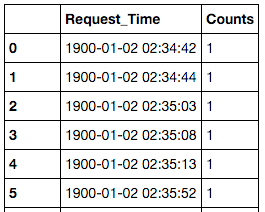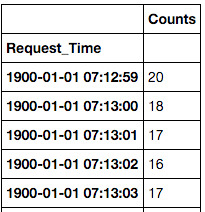In this post I will attempt to explain how I used `Pandas` and `Matplotlib` to quickly generate server requests reports on a daily basis.

Problem To Be Solved: Generate a Scatter plot of the number of requests to a particular URL along with the 99th, 95th and 90th percentile of requests for the duration of a day.Breakdown:

1. Reading multiple server access log files.
2. Parsing the timestamp fields so that the graph can be scaled appropriately.
3. Aggregating the timestamp fields.
4. Calculating the Quantile values.
5. Generating the Scatter Plot.

In this post I will leave out the details related to point number 1 and will concentrate on the remaining points. Since this was a fairly mundane task and had to be done daily for a couple of weeks, I intended on automating the process as well as generating the scatter plot as quickly as possible.

### Parsing the timestamp fields so that the graph can be scaled appropriately

Let’s assume the input file contains only timestamps and the file has been read into a list using the following code.

``````lines = [line.rstrip('\n') for line in open(file_name)]
``````

Since the x-axis of the scatter plot will contain timestamp values, the `lines` `List` which currently contains string values, needs to be converted into a `List` of timestamp values. My initial instinct was to use an explicit `for` loop. In an effort to speed up my script I came across python’s built-in `map()` function and the concept of `List` comprehension which reduce the `for` loop overhead when the loop body is relatively simple, check here for more details. After a bit of benchmarking I settled on using the `List` comprehension method.

### Aggregating the datetime fields

In this aggregation step the goal was to perform a group-by on the timestamps in order to calculate the number of requests per second. To achieve this I used `Numpy` which is a package for scientific computing and `Pandas` which is a data analysis library. The `Series` and `DataFrame` objects from the `Pandas` library and the `ones()` method from the `Numpy` package are used to generate the data structure show in Figure 1, the code snippet below contains the details.

Note: I could have probably achieved the same result using only `Pandas`.

The `DataFrame` object is a tabular data structure with labeled rows and columns, you may think of it as an Excel Spreadsheet. After creating the `DataFrame` `df` you will end up with a structure as displayed below.Figure 1: Pandas DataFrame

The next step is to group-by the Request_Time column and sum up the counts, this is achieved by calling the `groupby` method on the `DataFrame` object.

This gives us an aggregated `DataFrame` as displayed below### Calculating the Quantile values

Calculating the quantile values on the aggregated `DataFrame` can be done by calling the `quantile()` method which returns a `DataFrame` containing the value and the `dtype`.

### Generating the Scatter Plot

The Scatter plot is generated using the `Matplotlib` library. Since we are plotting timestamp values on the x-axis we will use the `plot_date()` method.

The x-axis contains the timestamp values, the y-axis contains the request counts, `xdate=True` indicates the x-axis contains date values.

Note: The `Matplotlib` library contains extensive documentation on all it’s API’s as well as a vast array of examples, hence I will refrain from going into more details in this post, you may check this link for more details.

The snippet below contains code related to rendering the Scatter Plot. I have taken a very simplistic and naive approach since it was good enough for my requirement.

### Summary

In this post we have seen how:

• `List` comprehension can give us better performance over an explicit `for` loop when the loop body is relatively simple. Please check here for more details on the topic.
• The `Pandas` library can be used to calculate aggregates and quantiles. We used the `Series` and `DataFrame` objects which are the core data structures of the `Pandas` library to achieve this.
• Generate a Time-Series Scatter Plot using the `Matplotlib` library.